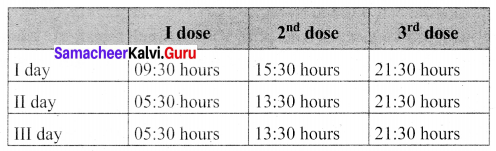## Tamilnadu Samacheer Kalvi 6th Maths Solutions Term 2 Chapter 2 Measurements Ex 2.3

Miscellaneous Practice Problems

Question 1.
Two pipes whose lengths are 7 m 25 cm and 8 m 13 cm joined by welding and then a small piece 60 cm is cut from the whole. What is the remaining length of the pipe?
Solution: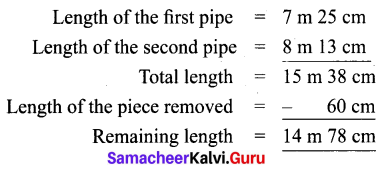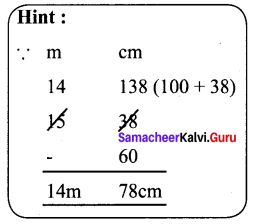Question 2.
The saplings are planted at a distance of 2 m 50 cm in the road of length 5 km by saravanan. If he has 2560 saplings, how many saplings will be planted by him? how many saplings are left?
Solution:
Distance between two saplings = 2 m 50 cm = 250 cm
Total length of the road = 5000 m = 500000 cm

Question 3.
Put ✓ a mark in the circles which adds up to the given measure.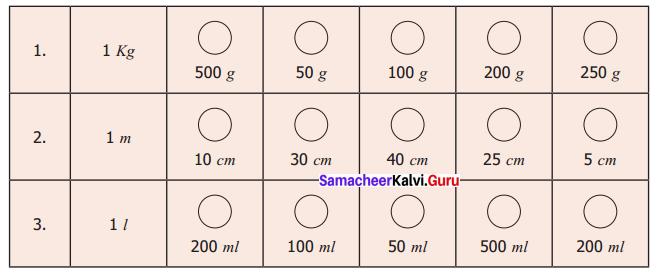Solution: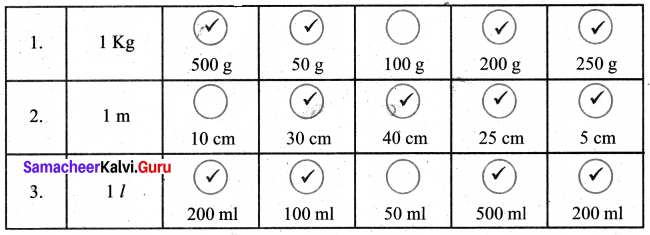Question 4.
Make a calendar for the month of February 2020 (Hint: January 1st 2020 is Wednesday)
Solution:
Given Jan 1st 2020 is Wednesday.
1st + 7 = 8th Wednesday
8th + 7 = 15th Wednesday
15th + 7 = 22nd Wednesday
22nd + 7 = 29th Wednesday
Jan = 30th Thursday
Jan 31st Friday
Feb 1st Saturday
February has 29 days as 2020 is a leap year.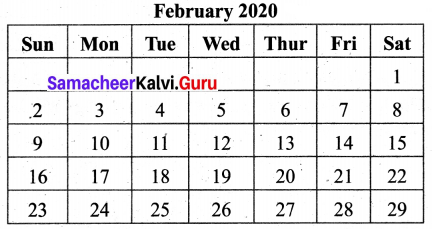Question 5.
Observe and Collect the data for a minute: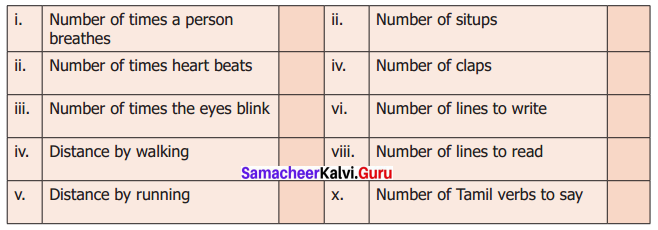Solution: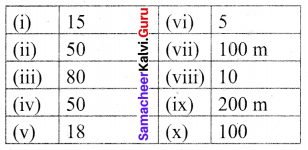Challenge Problems

Question 6.
A squirrel wants to eat the grains quickly. Help the squirrel to find the shortest way to reach the grains. (Use your scale to measure the length of the line segments)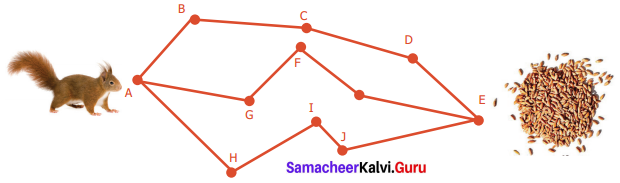Solution:
The squirrel is in A and the grains are at E
The ways are (i) A → B → C → D → E
(ii) A → G → F → K → E
(iii) A → H → I → J → E
Distance
(i) AB + BC + CD + DE = 2 cm + 2.5 cm + 2.5 cm + 2 cm = 9 cm
(ii) AG + GF + FK + KE = (2.6 + 1.7 + 1.8 + 3) cm = 9.1 cm
(iii) AH + HI + IJ + JE = 3 cm + 2.3 cm + 1 cm + 3.2 cm = 9.5 cm.
1st way ABCDE is the shortest one.Question 7.
A room has a door whose measures are 1 m wide and 2 m 50 cm high.
(i) Can we make a bed of 2 m and 20 cm length and 90 cm wide into the room?
Solution:
Measures of the door length 2 m 50 cm width and lm (100 cm)
(i) Measures of the bed 2 m 20 cm width and 90 cm
Measures of the bed < measures of the door
∴ Yes, we can take the bed into the room.

Question 8.
A post office functions from 10 a.m to 5.45 pm with a lunch break of 1 hour. If the post office works for 6 days a week. Find the total duration of working hours in a week.
Solution:
Working hours in a day = 6 hrs 45 min
= (6 × 60 min) + 45 min
= (360 + 45) min
= 405 min
Total duration of working hours in a week
= 6 × 405 min
= 2430 min
= $$\frac{2430}{60}$$
= $$\frac{810}{20}$$
= 40 $$\frac{1}{2}$$
= 40 hours 30 minutes

Question 9.
Seetha wakes up at 5.20 a.m. She spends 35 minutes to get ready and travels 15 minutes to reach the railway station. If the train departs exactly at 6 : 00 a.m, will Seetha catch the train?
Solution:
Time of wake up = 5.20 a.m = 5 hour 20 minutes
Time spends = 35 minutes
Then the time = 5 hour 55 minutes
Travelling time to reach railway station = 15 minutes
Now the time will be = 5 hours 70 minutes
= 5 hours (60 + 10) minutes
= 5 hours + (1 hour 10 minutes)
= 6 hours 10 minutes
= 6.10 a.m.
The departure time of the train to get ready = 6.0 a.m.
∴ She will not be able to catch the train.

Question 10.
A doctor advised Vairavan to take one tablet every 6 hours once in the 1st day and once every 8 hours on the 2nd and 3rd day. If he starts to take 9.30 a.m first dose. Prepare a time chart to take the tablet in railway time.
Solution:
The first dose is taken at 9.30 a.m. = 09:30 hours
Duration of every dose in 1st day = 6 hours
Duration of every dose in 2nd and 3rd day = 8 hours
Time Chart.